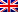# Cross-entropy method

Contenus

## Cross-entropy method

Cross-entropy method was developed as an efficient estimation technique for rare event probabilities in discrete event simulation systems and has been adapted for use in optimization. The name of the technique comes from the Kullback-Leibler's cross-entropy method for measuring the amount of information (bits) needed to identify an event from a set of probabilities.

The algorithm's information processing strategy consists of sampling the space of the problem and approximating the distribution of the good solutions. This is achieved by assuming a distribution of the space of the problem (such as Gaussian), sampling the domain of the problem by generating candidate solutions using the distribution, and updating the distribution according to the best candidate solutions discovered. Samples are constructed in stages (one component at a time) based on the summarized distribution of the correct solutions. As the algorithm progresses, the distribution becomes more refined until it focuses on the domain or scope of the optimal solutions in the domain.

The following algorithm describes the cross-entropy method to minimize a cost function.

The cross-entropy method has been adapted to combinatorial optimization problems, although it has been applied to continuous function optimization as well as to simulation problems containing a lot of noise.

An alpha parameter (a) or a learning rate in [0; 1] is usually set to a high level, such as 0.7. A smoothing function can be used to further control updates to the distribution (s) of the samples in the definition space. For example, in the optimization of a continuous function, a parameter can replace (a) for the update of the standard deviation, calculated at time t as BB (1-1 / t) ^ q, where B is initially set in [0.8; 0.99] and q is a small integer in [5; 10].FRFRENES